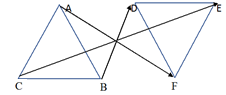In the verge of coronavirus pandemic, we are providing FREE access to our entire Online Curriculum to ensure Learning Doesn't STOP!

# Ex.7.1 Q4 Congruence of Triangles - NCERT Maths Class 7

Go back to  'Ex.7.1'

## Question

If $$ΔDEF ≅ ΔBCA$$, write the part(s) of $$ΔBCA$$ that correspond to

$$\left( \text{i} \right)\,\,\angle E\\\left( \text{ii} \right)\text{ }\overline{EF}~\\\left( \text{iii} \right)\,\,\angle F~\\\left( \text{iv} \right)\overline{\text{ }DF}.$$

Video Solution
Congruence Of Triangles
Ex 7.1 | Question 4

## Text Solution

What is the known?

Two $$ΔDEF$$ and $$ΔBCA$$ which are congruent.

What is the unknown?

The part(s) of $$ΔBCA$$ that correspond to different angles and sides.

Reasoning:

This question is based on the concept of congruence of triangles. As it is given in the question two triangles $$DEF$$ and $$BCA$$ are congruent. We can place triangle $$DEF$$ on $$BCA$$ such that $$D$$ falls on $$B$$, $$F$$ falls on $$A$$, $$F$$ falls on $$A$$ and $$D$$ falls on $$C$$.

Steps:

Since, $$ΔDEF ≅ ΔBCA$$

For better understanding of this concept it is best to visualize it with the help of figure.\begin{align}&\left( \text{i} \right)\;\;\angle E\leftrightarrow \angle C\\& \left( \text{ii} \right)\;\overline{EF}\leftrightarrow \overline{CA}\\&\left( \text{iii} \right)\angle F\leftrightarrow\angle A \\ & \left( \text{iv} \right)\overline{DF}\leftrightarrow \overline{BA} \\ \end{align}

Learn from the best math teachers and top your exams

• Live one on one classroom and doubt clearing
• Practice worksheets in and after class for conceptual clarity
• Personalized curriculum to keep up with school